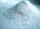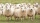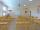Blood

In human body the blood is about 7.3% body weight. How many kilograms of blood is in the human body with weight 109 kg?

Result

x =  7.957 kg

Solution:Leave us a comment of this math problem and its solution (i.e. if it is still somewhat unclear...):Be the first to comment!To solve this verbal math problem are needed these knowledge from mathematics:

Need help calculate sum, simplify or multiply fractions? Try our fraction calculator. Our percentage calculator will help you quickly calculate various typical tasks with percentages.

Next similar math problems:

1. PersonsPersons surveyed:100 with result: Volleyball=15% Baseball=9% Sepak Takraw=8% Pingpong=8% Basketball=60% Find the average how many like Basketball and Volleyball. Please show your solution.
2. Apples 14Maya bought 18 apples. She used 50% of the apples to make apple pies and 1/3 of the apples was mixed with other fruits to make salad. How many apples were left?
3. The ballThe ball was discounted by 10 percent and then again by 30 percent. How many percent of the original price is now?
4. Price increase 2xIf two consecutive times we increase the price of the product by 20%, how many % is higher final price than the original?
5. CacaoCacao contains 34% filling. How many grams of filling are in 130 g cacao.
6. Frameworks is badCalculate how many percent will increase the length of an HTML document, if any ASCII character unnecessarily encoded as hexadecimal HTML entity composed of six characters (ampersand, grid #, x, two hex digits and the semicolon). Ie. space as: &#x20;
7. PercentsHow many percents is 900 greater than the number 750?
8. Highway repairThe highway repair was planned for 15 days. However, it was reduced by 30%. How many days did the repair of the highway last?
9. SummerjobThe temporary workers planted new trees. Of the total number of 500 seedlings, they managed to plant 426. How many percents did they meet the daily planting limit?
10. Red diplomasHe numbers of students with honors in 2013 and 2014 are in ratio 40:49. How big is the year-on-year percentage increase?
11. Bath saltsBath salt A weighs 900 grams and costs 36,40 CZK. Bath Salts B weighs 1000 grams, but costs 85 CZK. How much is a bath salt A cheaper than B one?
12. Percentage 1052 shorts and missed 13. Calculate percentage
13. On a farmOn a farm, there are 90 rabbits, 700 sheep, 300 cattle and 500 pigs. What percentage of the total number of animals are rabbits?
14. Percentages52 is what percent of 93?
15. Classroom 4In a class of 36 pupils, 2/3 are girls. How much it is in a class girls and boys?
16. Liters od milkThe cylinder-shaped container contains 80 liters of milk. Milk level is 45 cm. How much milk will in the container, if level raise to height 72 cm?
17. Profit gainIf 5% more is gained by selling an article for Rs. 350 than by selling it for Rs. 340, the cost of the article is: